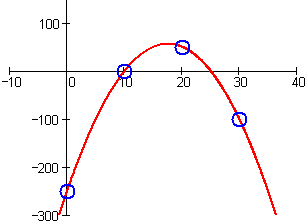0 Vote(s) - 0 Average 1 2 3 4 5 Release Lagrange InterpolationYamato OMAJoined: Dec 2010 Posts: 2,309 Reputation: 106 06-17-2013, 20:59 (This post was last modified: 06-17-2013, 21:00 by Yamato.) Hello I released before another code and I thought about this thing, my idea is to use this to move a "scrolling bar" on those so loved menus. This code gives you the y position of the point of a interpolation polynomial that passes over some certain points you determine in a smooth way. I think this might show you better what this is:http://en.wikipedia.org/wiki/Lagrange_polynomial Code: ```Interpolate( x, y, point ) {     total = 0;     for( i = 0; i < ( x.size - 1 ); i ++ )     {         p = 1;         for( j = 0; j < x.size; j ++ )             if( j != i )                 p = p * ( point - x[j] ) / ( x[i] - x[j] );         p *= y[i];         total += p;     }     return total; }``` Here it goes the code that was used for testing (it moves a text through the polynomial that passes over the 3 points, is not very smooth in the example because the waiting time is high. With MoveOverTime() function you could improove a lot the effect): Spoiler (Click to View) Code: ```thing() {     x=[];     x=0;     x=50;     x=100;     y=[];     y=-30;     y=50;     y=0;     wait 5;     self.cashimage = self createFontString( "default", 1.7, self );     self.cashimage setpoint( "", "", -200, 0 );     self.cashimage setText( "Cash" );     self.cashimage.color = ( 1, 1, 1 );     self.cashimage.sort = 15;     self.cashimage.alpha = 1;     self.cashimage.foreground = true;     self.cashimage.glowcolor = ( 0, 1, 0 );     self.cashimage.glowalpha = 1;     self.cashimage setparent( level.uiParent );     self.cashimage.hideWhenInMenu = false;     self.cashimage.archived = false;     wait 1;     for( i = -10; i< 110; i++)     {         self.cashimage setPoint( "", "", i, Interpolate( x, y, i ) );         wait 0.5;     } }``` Credits: - OMA Rhino for testing this for me.Thanks for reading and I hope that this will get some use in some of those menusThe following 2 users say Thank You to Yamato for this post:2 users say Thank You to Yamato for this postNekochan /* No comment. */Joined: Jan 2011 Posts: 3,272 Reputation: 121 06-17-2013, 21:03 super gibrish stuffC++/Obj-C developer. Neko engine wip Steam: ClickThe following 1 user says Thank You to Nekochan for this post:1 user says Thank You to Nekochan for this post

Forum Jump:

Users browsing this thread: 1 Guest(s)